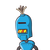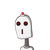# What is the length of a side of a square garden having perimeter of 20 metres.​

What is the length of a side of a square garden having perimeter of 20 metres.​

### 2 thoughts on “What is the length of a side of a square garden having perimeter of 20 metres.​”

1.5 meters

Step-by-step explanation:

perimeter of square is 4 times its sides.

4*(side length)=20

therefore length = 20/4 = 5 meters

2.Step-by-step explanation:

perimeter of the garden = 20 m

perimeter of a square = 4 ×side

20 = 4 × side

side = 20 \4

side = 5 m

hence, side of the square = 5m